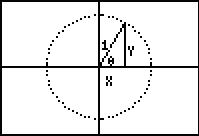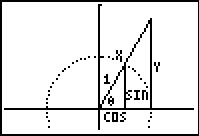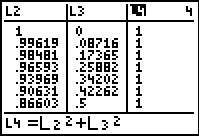••• ##### Device
• TI-83 Plus Family
• TI-84 Plus
• TI-84 Plus Silver Edition
•TI-84 Plus C Silver Edition
•TI-84 Plus CE
• ##### Software

TI Connect™
TI Connect™ CE

# SA and NT: Trigonometric Identities

by Texas Instruments#### Overview

1.4 Trigonometric Functions

Students will verify, prove, and explore trigonometric identities symbolically, numerically, and graphically.

#### Key Steps

•Students will use the VERITRIG program to help prove the two trigonometric identities. They will use the Text tool to label a triangle drawn within the unit circle. Then students will write x and y in terms of cosθ and sinθ to prove the identity cos2θ + sin2θ = 1.

•Students then prove the identity sec2θ = 1 + tan2θ using similar triangles and ratios.

•After proving the identities, students can use the cosine and sine values of the angles in the unit circle to prove that the proof holds for each identity.